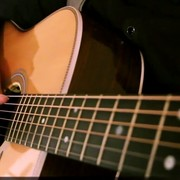{meta: 原调: 1=D} {meta: 选调: 1=D} {meta: Capo=0} {comment: 前奏} [D] [F#m] [G] [A] [D] [F#m] [G] {comment: 主歌 A} [D]心跳乱了节[F#m]奏 [G]梦也不自[A]由 [D]爱 是个绝对[F#m]承诺 不[G]说 沉到一千[A]年以后 [Bm]放任无奈 [A]淹没尘埃 [G]我在废墟之中守着[D]你走[A]来 喔 [Bm]我的泪光 [A]承载不了 [G]喔 所有一切你要的[A]爱 {comment: 副歌} {start_of_chorus} 因为在 [D]一千年以[A]后 世界[Bm]早已没有[A]我 无法[G]深情挽着[Bm]你的手 浅[G]吻着你额[A]头 别等到 [D]一千年以[A]后 所有人[Bm]都遗忘了[A]我 那时[G]红色黄昏[Bm]的沙漠 能有[G]谁 解开[Gm]缠绕千年的[D]寂寞 {end_of_chorus} {comment: 前奏/间奏} [D] [F#m] [G] [A] [D] [F#m] [G] {comment: 主歌 A} [A] 呜噢噢噢噢 [Bm]放任无奈 [A]淹没尘埃 [G]我在废墟之中守着[D]你走[A]来 喔 [Bm]我的泪光 [A]承载不了 [G]喔 所有一切你要的[A]爱 {comment: 副歌} {start_of_chorus} 因为在 [D]一千年以[A]后 世界[Bm]早已没有[A]我 无法[G]深情挽着[Bm]你的手 浅[G]吻着你额[A]头 别等到 [D]一千年以[A]后 所有人[Bm]都遗忘了[A]我 那时[G]红色黄昏[Bm]的沙漠 能有[G]谁 解开[Gm]缠绕千年的[D]寂寞 嗷嗷[G]嗷 [Gm]缠绕千年的~~~~~寂[D]寞 {end_of_chorus} {comment: 前奏/间奏} [F#m] [G] [A] [D] [F#m] [G] [D] {title:一千年以后} {artist:JJ} {author:李星宇}